# Unity3D新手教学，让你十二小时，从入门到掌握！（一）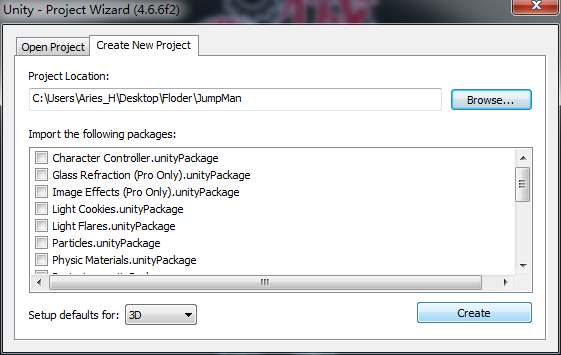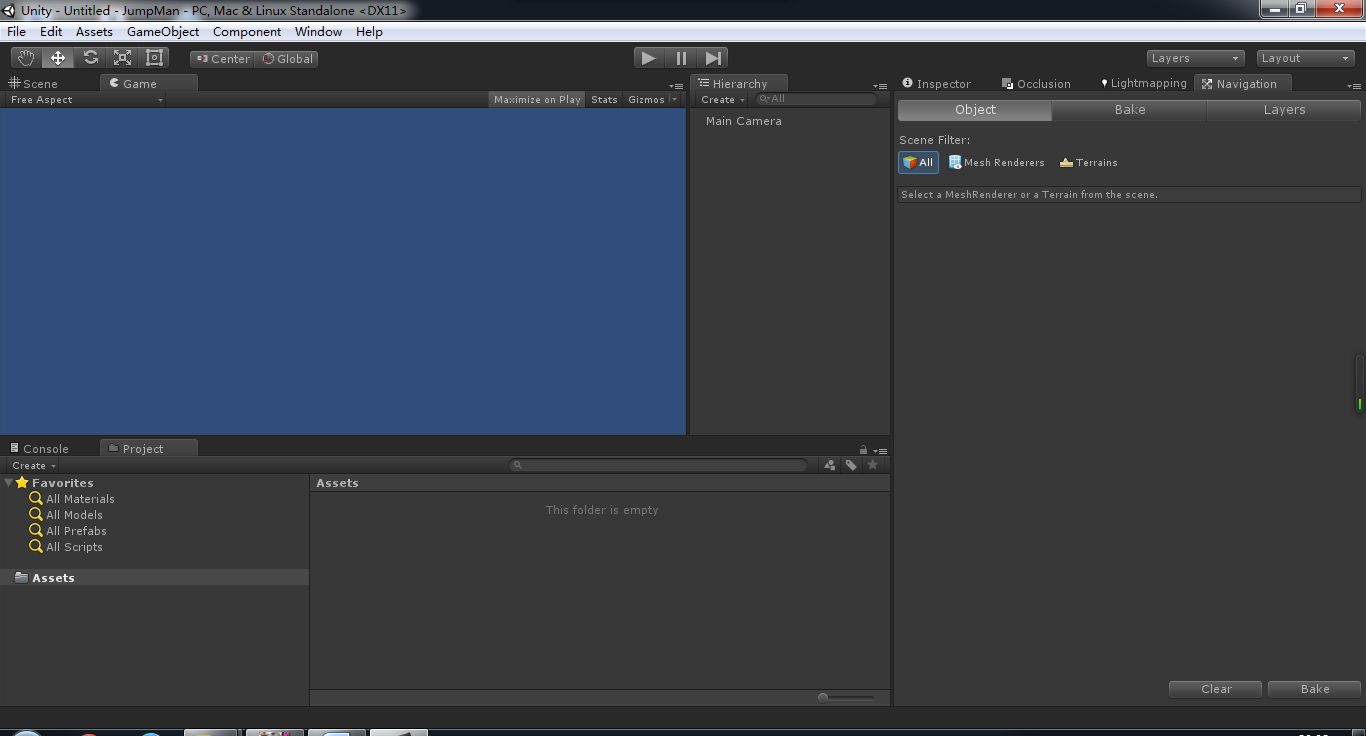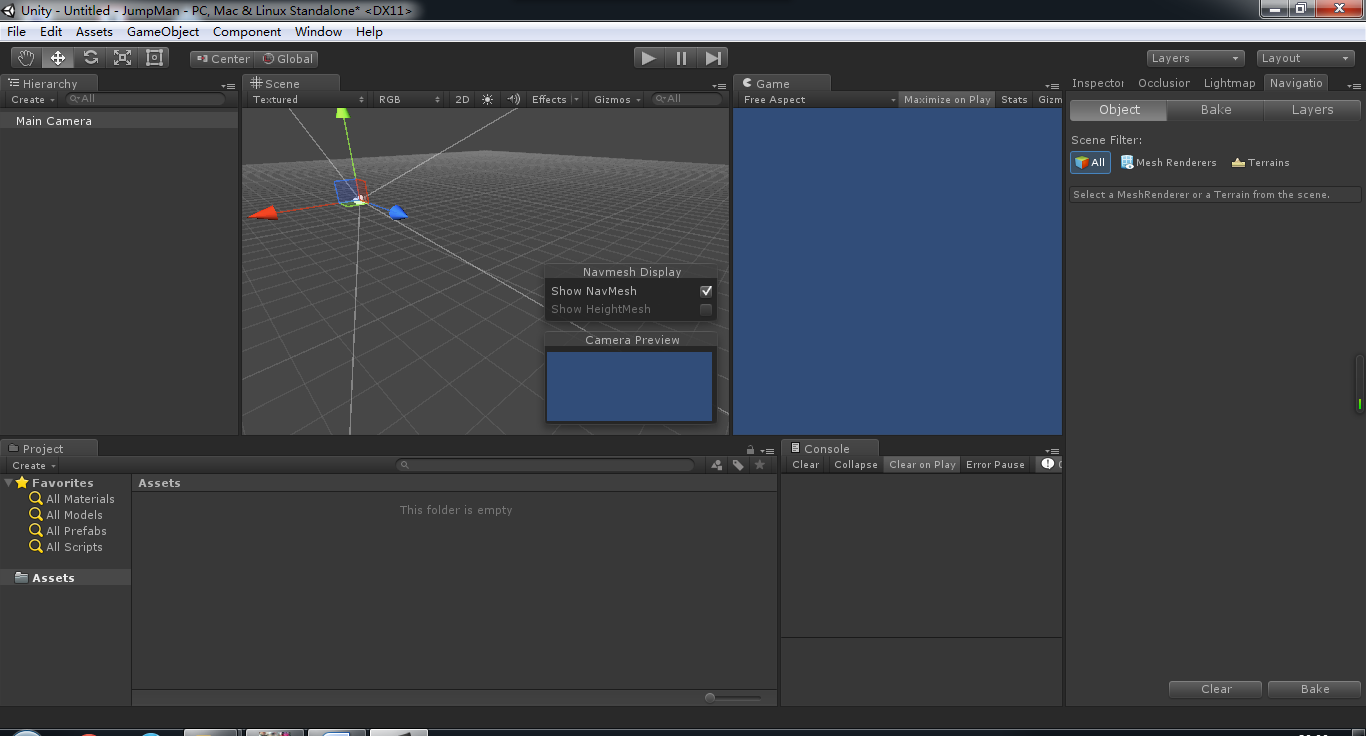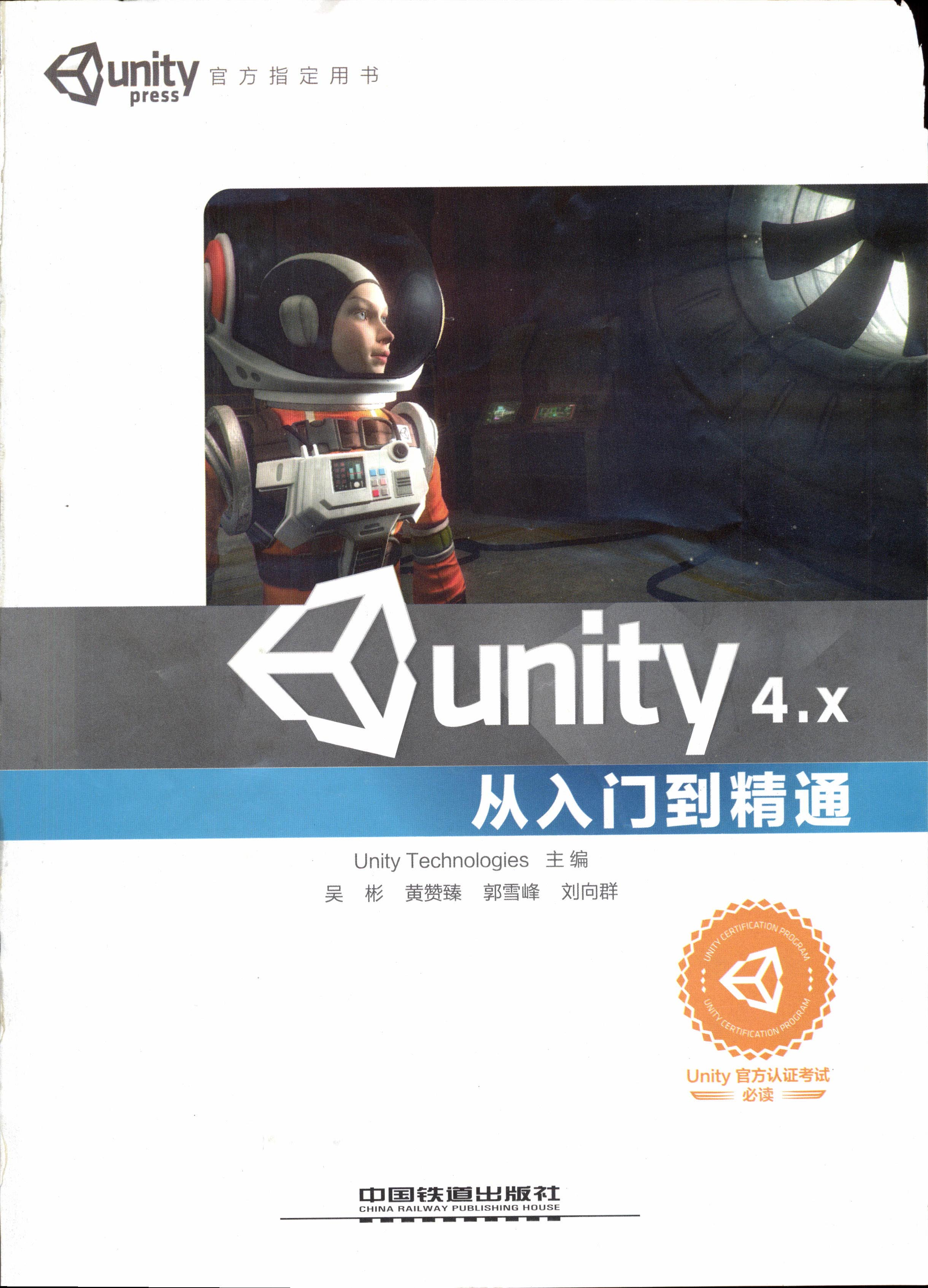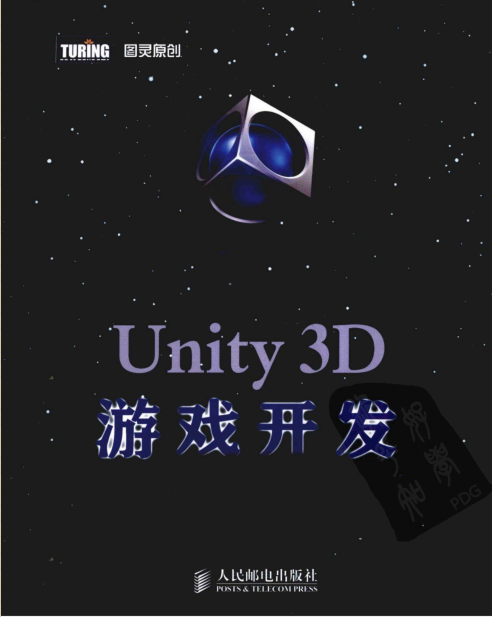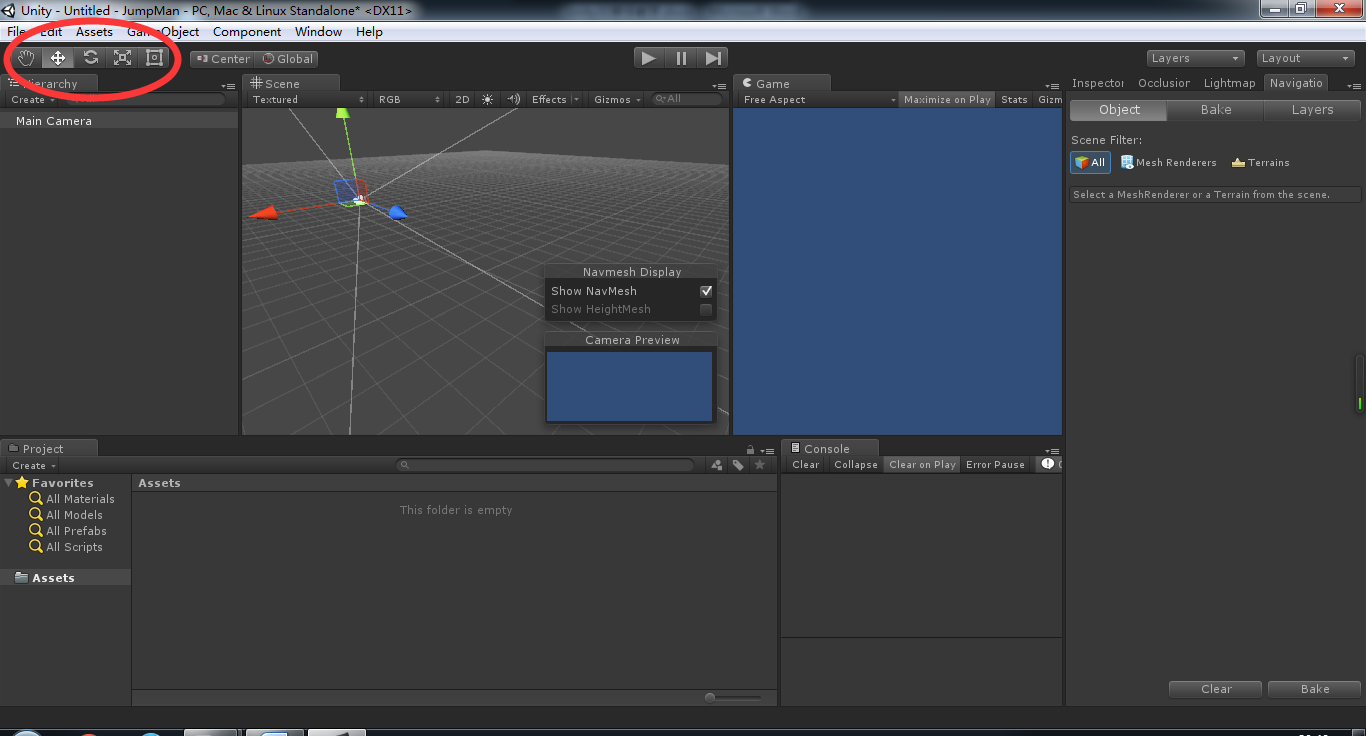Q：调整视图
W：移动工具
E：旋转工具
R：缩放工具
T：选择工具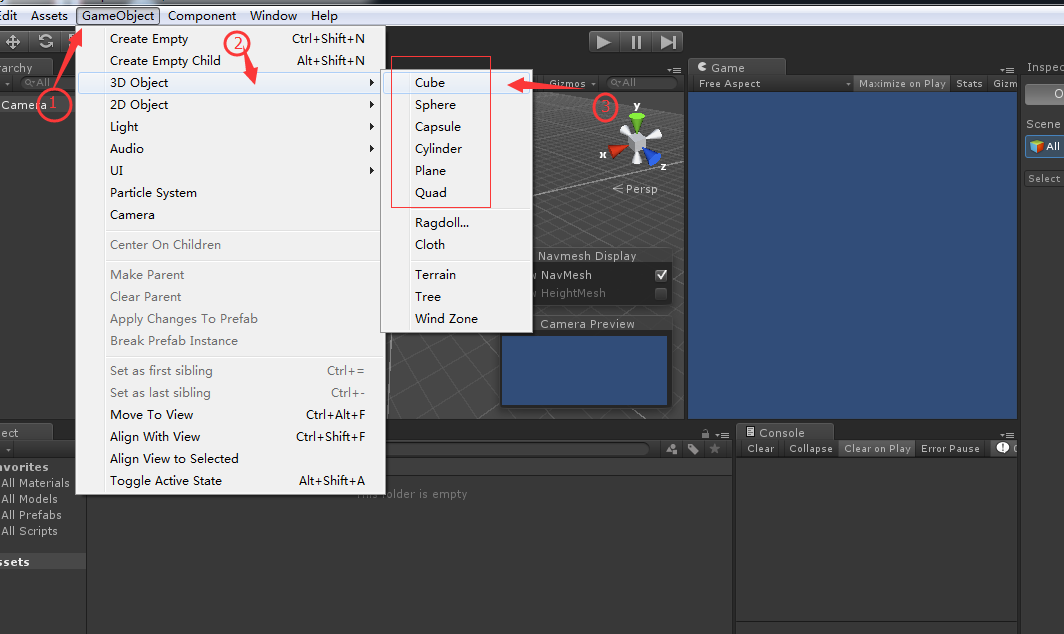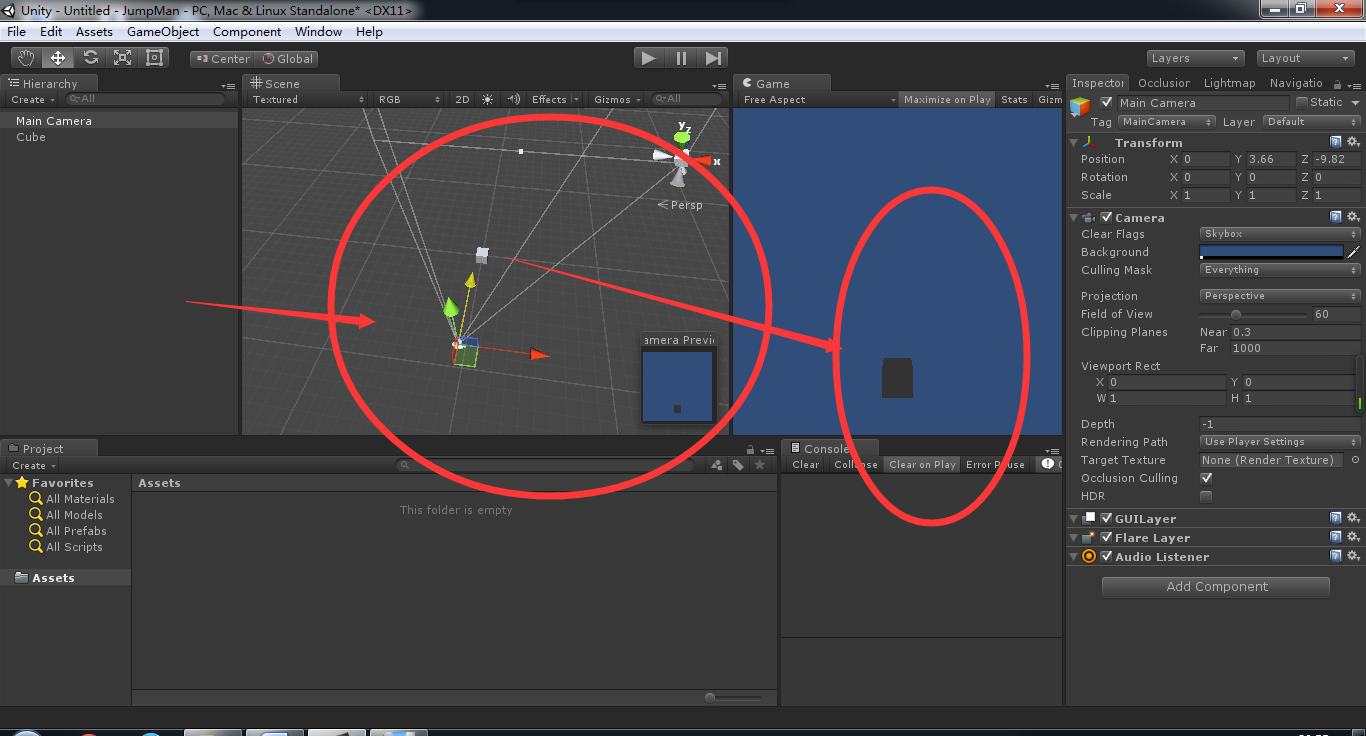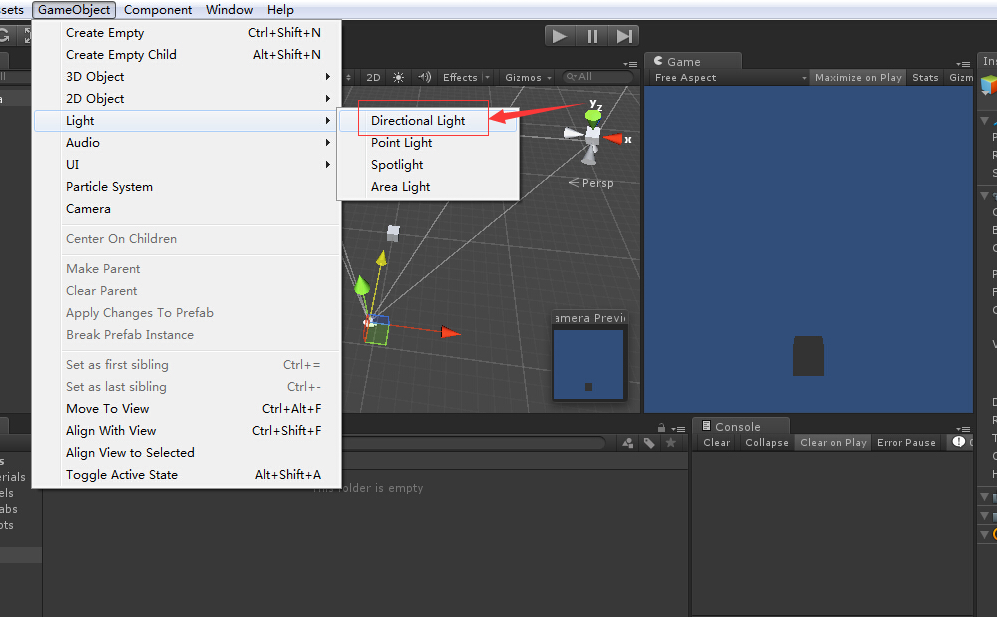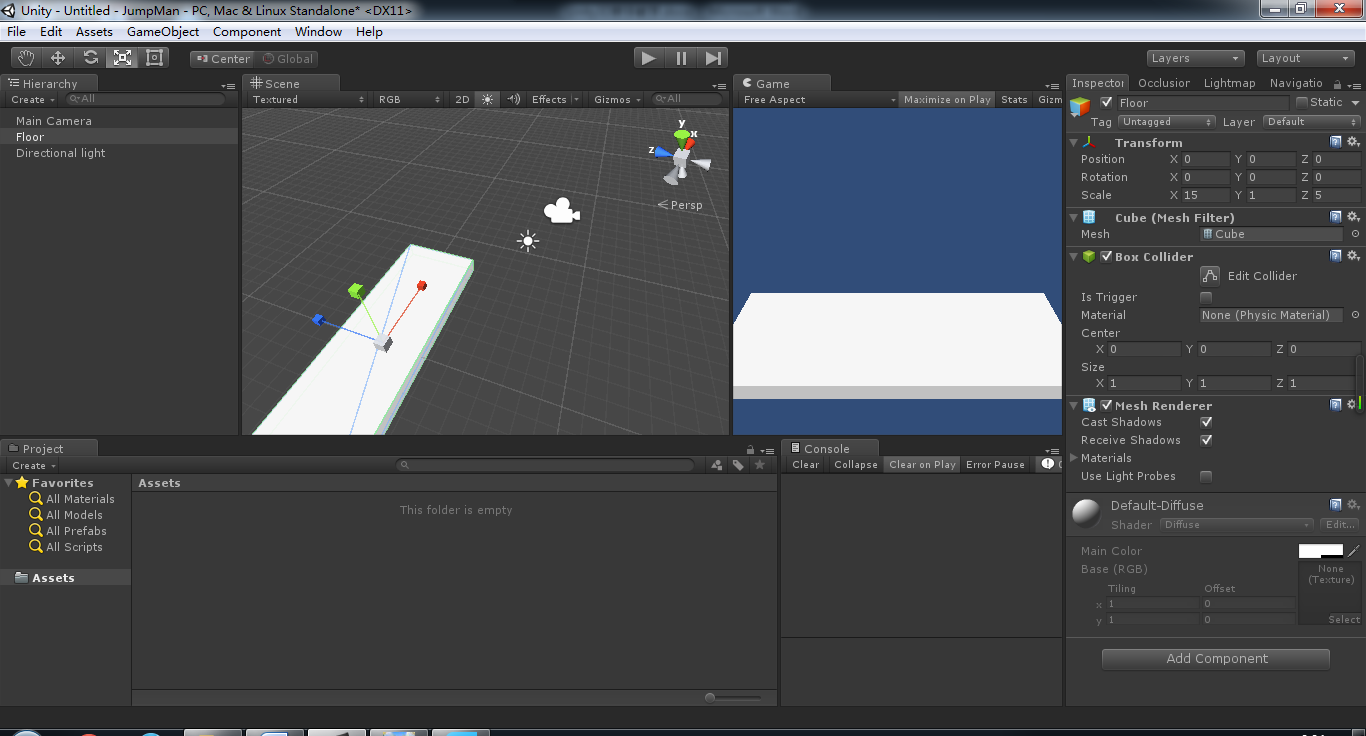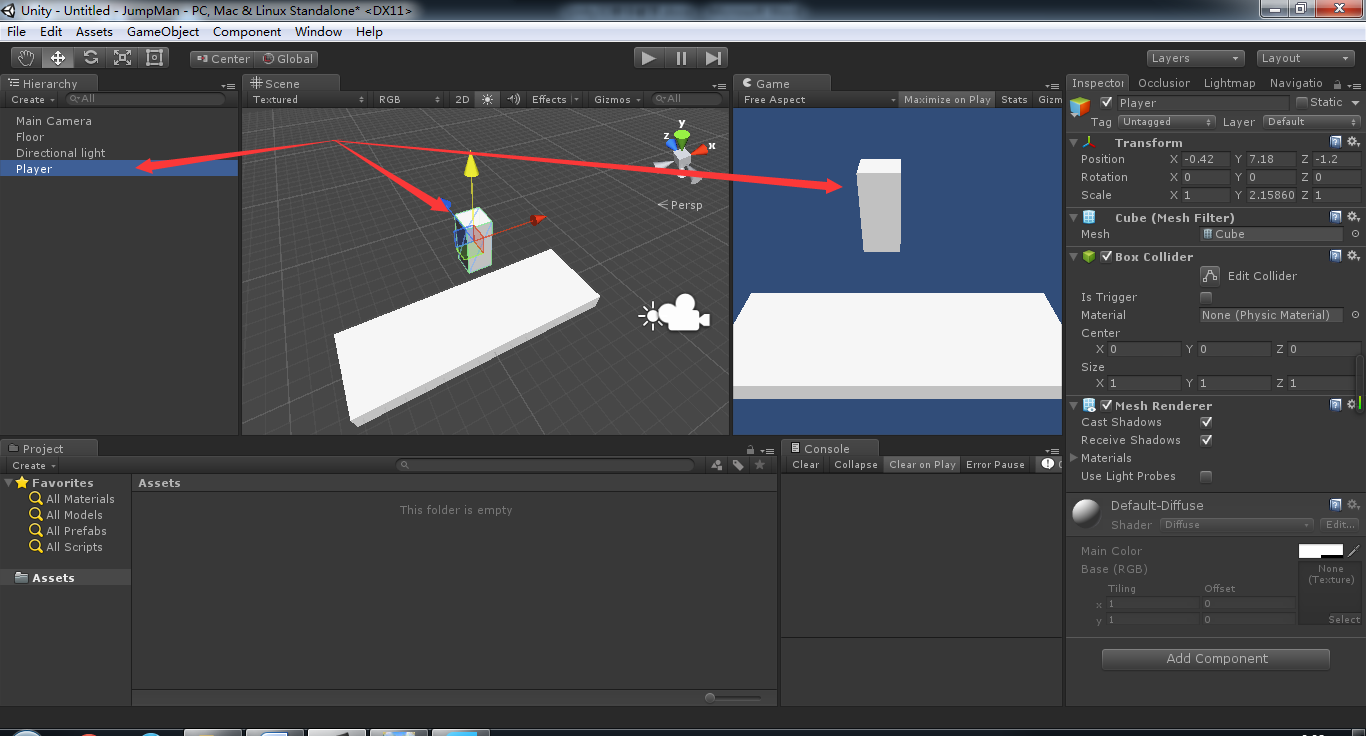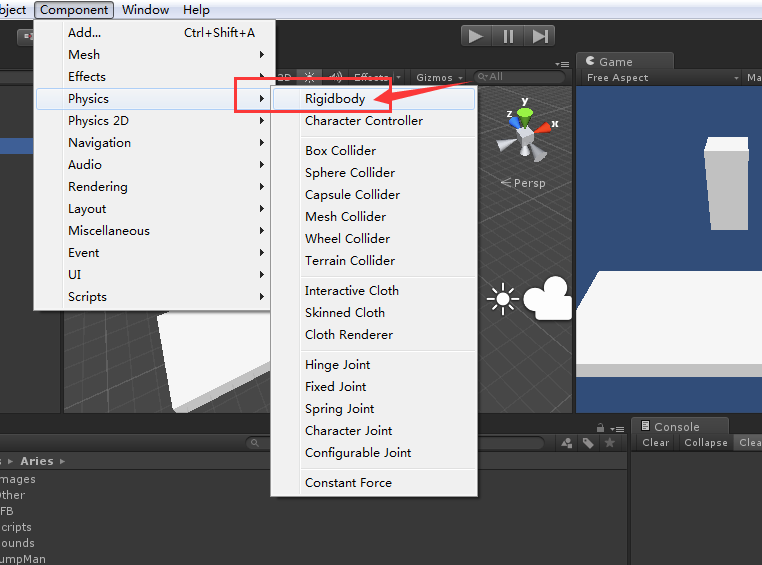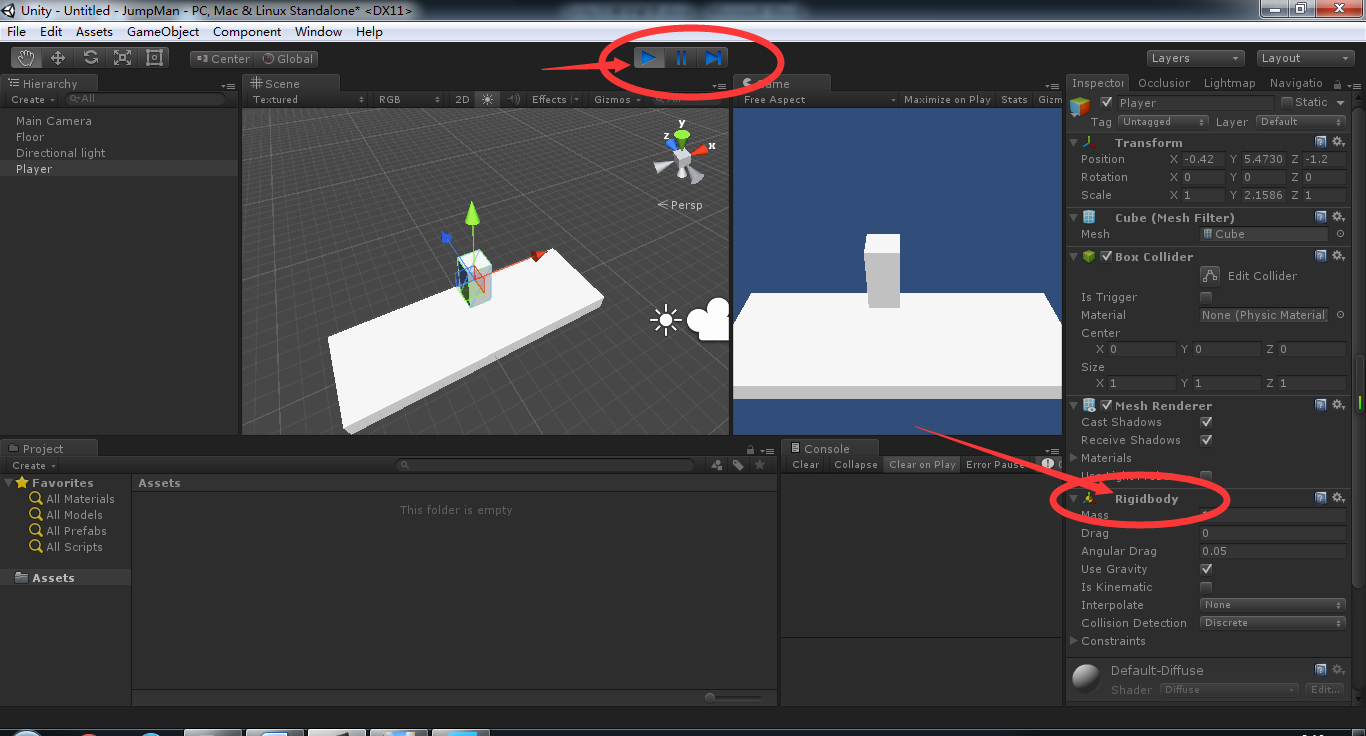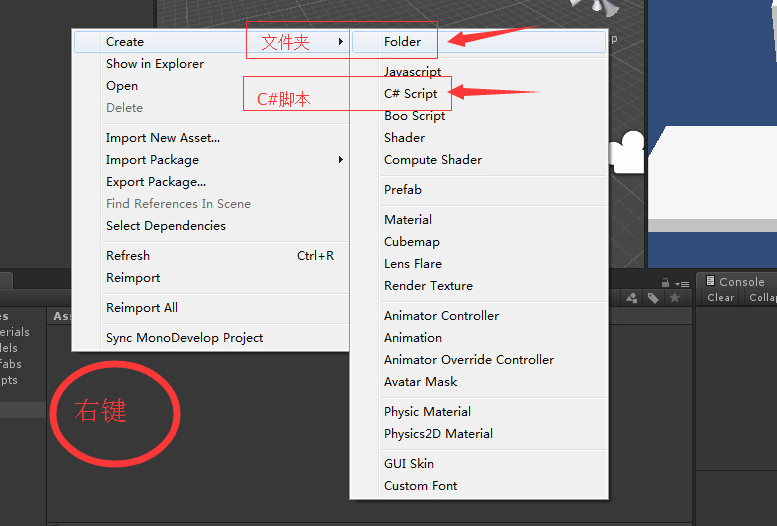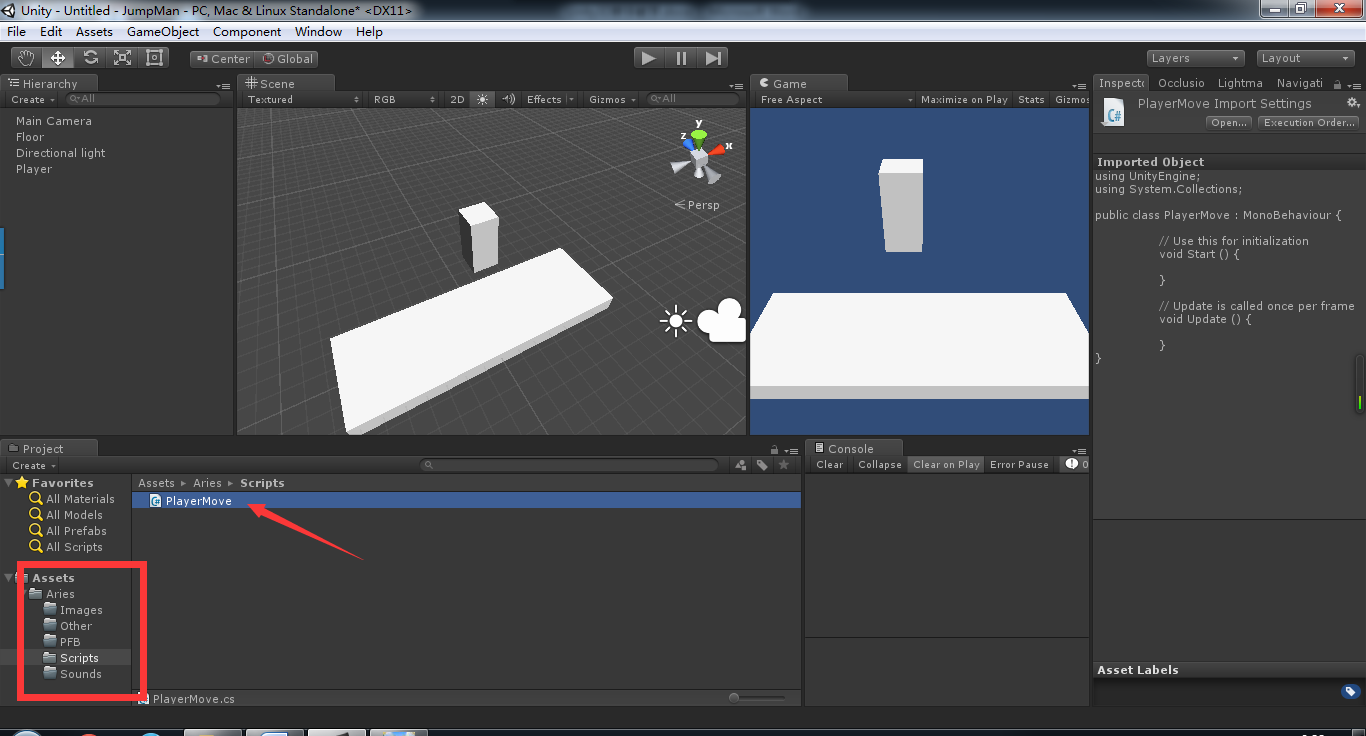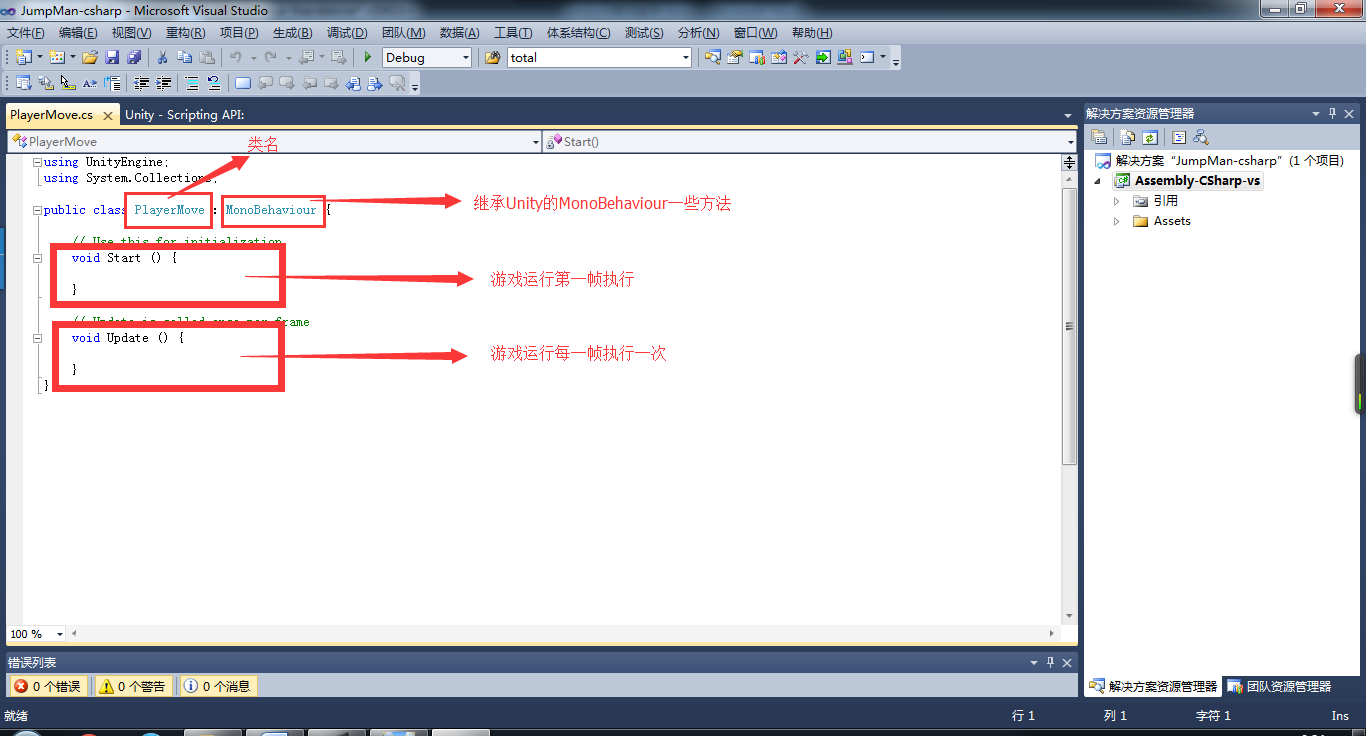public class PlayerMove : MonoBehaviour {

//定义移动的速度
public float MoveSpeed = 2f;
//定义旋转的速度
public float RotateSpeed = 0.01f;

void Start () {

}

void Update () {

//如果按下W或上方向键
if(Input.GetKey(KeyCode.W)||Input.GetKey(KeyCode.UpArrow))
{
//以MoveSpeed的速度向正前方移动
this.transform.Translate(Vector3.forward*MoveSpeed*Time.deltaTime);
}
if (Input.GetKey(KeyCode.S) || Input.GetKey(KeyCode.DownArrow))
{
this.transform.Translate(Vector3.back * MoveSpeed*Time.deltaTime);
}

//如果按下A或左方向键
if (Input.GetKey(KeyCode.A) || Input.GetKey(KeyCode.LeftArrow))
{
//以RotateSpeed为速度向左旋转
this.transform.Rotate(Vector3.down * RotateSpeed);
}
if (Input.GetKey(KeyCode.D) || Input.GetKey(KeyCode.RightArrow))
{
this.transform.Rotate(Vector3.up * RotateSpeed);
}

}
}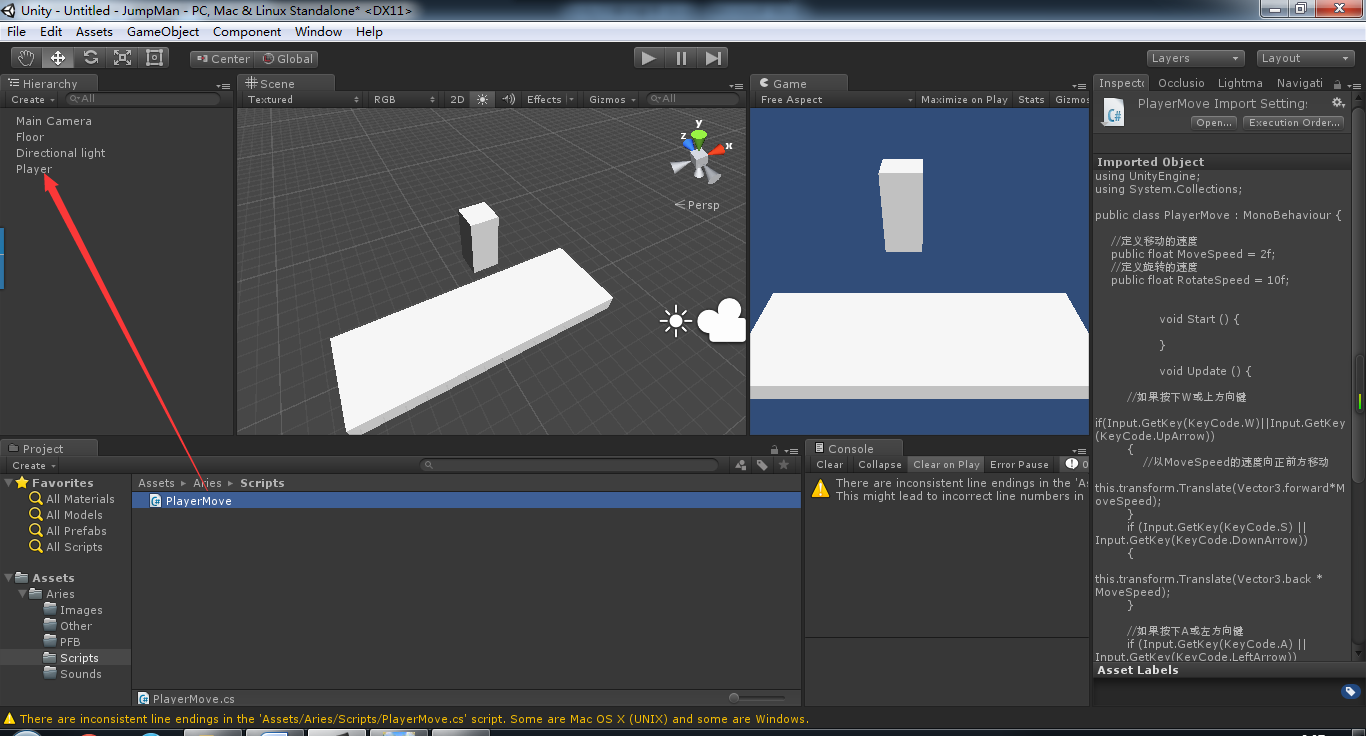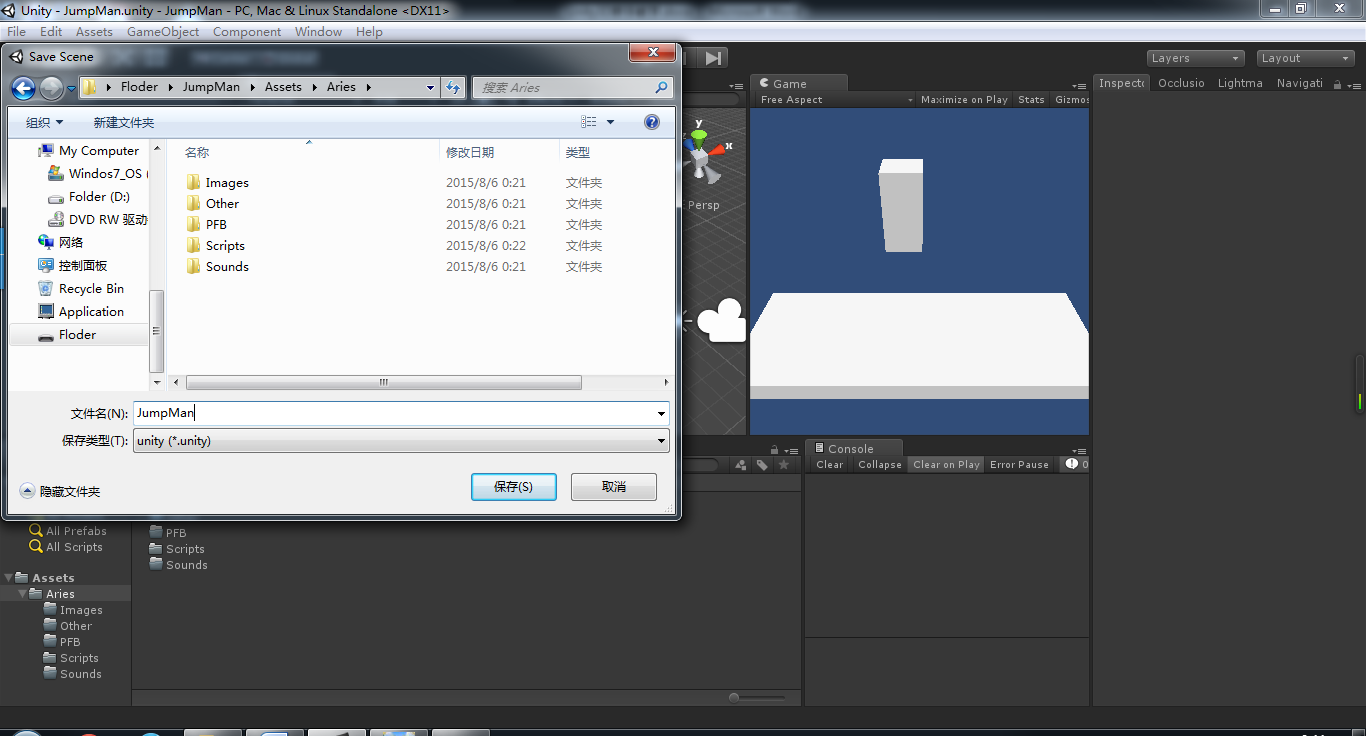QQ：531193915
E_Mail：15210411296@163.com